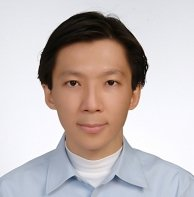# Wei-Chuan Wang

Associate Professor

General Education Building 509
Tel: 886-82-313912
Fax:886-82-313729
E-mail: wangwc@email.nqu.edu; wangwc72@gmail.com

### Education:

Ph.D. Department of Applied Mathematics , National Sun Yat-sen University, 2010
Department of Applied Mathematics , National Chiao Tung University, 1997
Department of Mathematics , Tunghai University, 1995

### Experience:

Post Doc. Researcher, National Changhua University of Education

### Research Interests:

Nonlinear differential equations, inverse problem

### Course Taught:

Calculus, Engineering Mathematics, General education courses related to Mathematics

### Publications

1. Wei-Chuan Wang and Yan-Hsiou Cheng* (2017, Jun). Sign-changing solutions to a class of nonlinear equations involving p-Laplacian. Rocky Mountain Journal of Mathematics, 47(3), 971-996.
1. Yan-Hsiou Cheng and Wei-Chuan Wang* (2017, Mar). Nodal properties for pLaplacian systems. Electronic Journal of Differential Equations, Vol. 2017 (2017), No. 87, pp.1-8.
1. K. Law, Wan-Zhen Wang and Wei-Chuan Wang* (2017, Jan). Sturm-Liouville properties for Atkinson’s semi-definite p-Laplacian eigenvalue problems. Mathematische Nachrichten, 290(1), 66-74.
1. Yan-Hsiou Cheng and Wei-Chuan Wang* (2016, May). Inverse nodal problems for the p-Laplacian with eigenparameter dependent energy functions. Boundary Value Problems, 2016:102 .
1. Yan-Hsiou Cheng, Chun-Kong Law, Wei-Cheng Lian and Wei-Chuan Wang (2015, Aug). An inverse nodal problem and Ambarzumyan problem for the periodic p-Laplacian operator with integrable potentials. Taiwanese Journal of Mathematics, 19(4), 1305-1316.
1. Wei-Chuan Wang* and Yan-Hsiou Cheng (2014, Mar). An inverse problem related to a half-linear eigenvalue problem. Boundary Value Problems, 2014:65.
1. Wei-Chuan Wang* and Yan-Hsiou Cheng (2014, Jan). On the existence of signchanging radial solutions to nonlinear p-Laplacian equations in R^n. Nonlinear Analysis Series A: Theory, Methods & Applications, 102(2014), 14-22. (SCI).
1. Yan-Hsiou Cheng, Wei-Cheng Lian and Wei-Chuan Wang (2014, Jan). The dual eigenvalue problems for p-Laplacian. Acta Mathematica Hungarica , 142 (1) (2014) , 132–151. (SCI).
1. Wei-Chuan Wang* (2013, Nov). Prufer substitutions on a coupled system involving the p-Laplacian. Electronic Journal of Differential Equations, Vol. 2013 (2013), No. 213, 1-9.
1. K. Law, V. Pivovarchik and Wei-Chuan Wang (2013, Oct). A polynomial identity and its application to inverse spectral problems in Stieltjes strings . Operators and Matrices , 7(3), 603-617.
1. Z. Chen, C.K. Law, W.C. Lian and Wei-Chuan Wang* (2013, Mar). Optimal upper bounds for the eigenvalue ratios of one-dimensional p-Laplacian. Proceedings of American Mathematical Society, 141(3), 883–893.
1. C. Lian, Wei-Chuan. Wang and Y.H. Cheng (2013, Mar). Existence of signchanging solutions for the nonlinear p-Laplacian boundary value problem. Analysis and Applications, Vol. 11, No. 2 (2013).
1. Wei-Chuan Wang*, Y.H. Cheng and W.C. Lian (2011, Jul). Inverse nodal problems for the p-Laplacian witheigenparameter dependent boundary conditions. Mathematical and Computer Modelling, 54, 2718-2724.
1. K. Law, Wei-Cheng Lian and Wei-Chuan Wang (2009, Sep). The inverse nodal problem and the Ambarzumyan problem for p-Laplacian. Proceedings of the Royal Society of Edinburgh, 139A, 1261-1273.